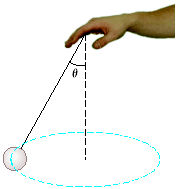# A 0.50 kg ball that is tied to the end of a 1.1 m light cord is revolved in a horizontal planewithHarlen Pritchard 2020-11-05 Answered

A 0.50 kg ball that is tied to the end of a 1.1 m light cord is revolved in a horizontal planewith the cord making a ${30}^{\circ }$ angle, with the vertical.a) Determine the ball speed.
b) If, instead, the ball is revolved so that its speed is 4.0 m/s,what angle does the cord make with the vertical?
c) If the cord can withstand a maximum tension of 9.1 N, what is the highest speed at which the ballcan move?

You can still ask an expert for help

• Questions are typically answered in as fast as 30 minutes

Solve your problem for the price of one coffee

• Math expert for every subject
• Pay only if we can solve itAubree Mcintyre
Part a)
$\frac{m{v}^{2}}{r}=T\mathrm{sin}\left(\theta \right)$
$mg=T\mathrm{cos}\left(\theta \right)$
$\mathrm{tan}\left(\theta \right)=\frac{{v}^{2}}{rg}$
${v}^{2}=rg\mathrm{tan}\left(\theta \right)$
but
$r=h\mathrm{tan}\left(\theta \right)$
${v}^{2}=h{g\left(\mathrm{tan}\left(\theta \right)\right)}^{2}$
${v}^{2}=1.1×9.8×{\left(\mathrm{tan}\left(30\right)\right)}^{2}$
$=3.5933$
$v=1.8956\frac{m}{s}$
Part(b):
${v}^{2}=h{g\left(\mathrm{tan}\left(\theta \right)\right)}^{2}$
${\left(\mathrm{tan}\left(\theta \right)\right)}^{2}=\frac{{4}^{2}}{1.1×9.8}$
$\theta =50.62$ degrees
Part (c):
If the cord can withstand a maximum tension of T=9.1N then the speed is given by
$\frac{m{v}^{2}}{r}=T\mathrm{sin}\left(\theta \right)$
${v}^{2}=\frac{rT\mathrm{sin}\left(\theta \right\}\left\{m\right\}}{}$
$=\frac{Th\mathrm{tan}\left(\theta \right)\mathrm{sin}\left(\theta \right)}{m}$
$=\frac{9.1×1.1×\mathrm{tan}\left(30\right)\mathrm{sin}\left(30\right)}{0.5kg}$
$v=2.404\frac{m}{s}$